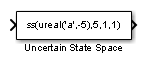# Uncertain State Space

•Libraries:
Robust Control Toolbox

## Description

The Uncertain State Space block lets you model parametric and dynamic uncertainty in Simulink®. The block accepts uncertain state space (`uss`) models or any model that can be converted to `uss`, such as `umat`, `ureal`, `umargin`, and `ultidyn` objects.

## Ports

### Input

expand all

For a single-input uncertain system, the input signal is a scalar. For multiple-input systems, combine the system inputs into a vector signal, using blocks such as:

### Output

expand all

For a single-output uncertain system, the output signal is a scalar. For multiple-output systems, the output signal is a vector. To split system outputs into scalar signals, use blocks such as:

## Parameters

expand all

Specify the uncertain model to simulate as `uss` object using one of the following:

• Function or expression that evaluates to an `uss` object. For example:

• `ss(ureal('a',-5),5,1,1)`

• `wt*input_unc`, where `input_unc` is an `ultidyn` object and `wt` and `input_unc` are defined in the MATLAB® workspace.

• Variable defined in the MATLAB workspace. For example, `unc_sys`, where you define `unc_sys` = `ss(ureal('a',-5),5,1,1)` in the workspace.

• Model of any type that can be converted to a `uss` model object. For example:

• LTI models (`tf`, `zpk` and `ss`)

• Uncertain matrix (`umat`)

• Uncertain real parameters (`ureal`)

• Uncertain dynamics (`ultidyn`, `umargin`)

When the block is in a model with synchronous state control (see the State Control (HDL Coder) block), you must specify a discrete-time model.

#### Programmatic Use

 Block Parameter: `USystem` Type: `uss` model, model that can be converted to `uss` Default: `ss(ureal('a',-5),5,1,1)`

The `uss` model that you specify in the Uncertain system variable (uss) parameter depends on uncertain variables such as `ureal` or `ultidyn` uncertainty. To simulate or linearize the uncertain model, the block must replace these uncertain variables with fixed values. Use this parameter to specify those fixed values for the next simulation or linearization. Use a structure whose fields are the names of the uncertain elements in the `uss` model and whose values are the substitute values of those elements. For example:

• If the `uss` model has uncertain real (`ureal`) parameters with names `a` and `b`, then setting this parameter to `struct('a',1,'b',3.5)` replaces `a` with 1 `b` with 3.5.

• If the `uss` model has dynamic uncertainty represented by a `ultidyn` element named `'delta'`, then setting this parameter to `struct('delta',tf(1,[1 1]))` replaces the uncertain dynamics with the specified transfer function.

To generate randomized values of uncertain variables for Monte Carlo simulation, use `ufind` and `usample`, as shown in the examples Simulate Uncertain Model at Sampled Parameter Values and Vary Uncertain Values Across Multiple Uncertain Blocks.

The default value `[]` sets all uncertain elements to their nominal values. Note that the nominal value of `ultidyn` uncertain dynamics is always 0, and the nominal value of `umargin` gain and phase uncertainty is always 1.

#### Programmatic Use

 Block Parameter: `UValue` Type: structure Default: `[]`

If the nominal value of the uncertain system you specify in the Uncertain system variable (uss) parameter has dynamics, you can use this parameter to specify initial values for those states. Specify the initial states as a vector having as many entries as there are states. The default value of `[]` initializes all states to 0.

#### Programmatic Use

 Block Parameter: `X0` Type: scalar, vector Default: `[]`

If the uncertain system contains some dynamic uncertainty (`ultidyn` or `umargin`), then you can use the Uncertainty value (struct or [] to use nominal value) parameter to replace that uncertainty with specific dynamics for simulation. Use the Initial states (uncertain dynamics) parameter to specify the initial state of these dynamics. Specify the initial states as a vector having as many entries as there are states. The default value of `[]` initializes all states to 0.

#### Programmatic Use

 Block Parameter: `uX0` Type: scalar, vector Default: `[]`

## Version History

Introduced in R2009b